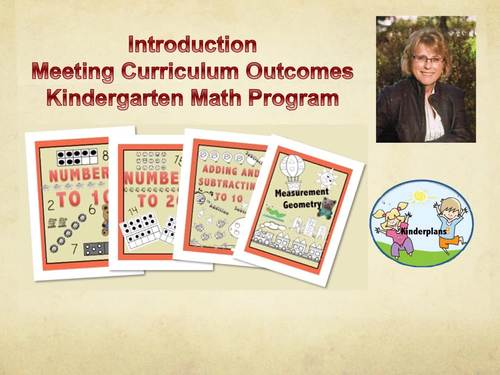# Kindergarten Math Curriculum Program;
K - 5th
Subjects
Resource Type
Standards
Formats Included
• Zip
\$23.13
List Price:
\$28.91
You Save:
\$5.78
Bundle
\$23.13
List Price:
\$28.91
You Save:
\$5.78
Bundle
Report this resource to TPT

### Description

This bundled package contains workbooks and activities to meet the Kindergarten common core curriculum outcomes. View the video for a brief overview of the program. You can also view each individual product for more information.

Counting and Cardinality

I can count forward starting at any number.

K.CC.A.2

I can write numbers 0-20 and show the correct amount of

objects for these numbers. K.CC.A.3

I know a number represents an amount of objects.

K.CC.B.4

When I count, I know each number I say represents one object.

K.CC.B.4aa

I know the last number I say is the number of objects, even if I

arrange them differently. K.CC.B.4b

I know when I count the number gets bigger.

K.CC.B.4c

I can count 20 things.

K.CC.B.5

I can tell if a group has greater than, less than or an equal amount.

K.CC.B.6

I can compare two printed numerals between 1-10.

K.CC.B.7

Math Operations in Base 10

I can tell what the height, width and weight of an object is. K.MD.A.1

I can compare the length, width and weight of two objects and

explain the difference. K.MD.A.2

I can sort objects into categories. K.MD.B.3

I can tell you the position of an object using words like above,

below, beside, in front of, behind, and next to.

K.G.A.1

I can name shapes no matter which way they are turned.

K.G.A.2

I know some shapes are 2-D and 3-D.

K.G.A.3

I can describe 2-D and 3-D shapes (corners, number of sides, etc.).

K.G.B.4

I can make 3-D shapes out of other objects.

K.G.B.5

I can decompose numbers by how many tens and ones there are.

K.NBT.A.1

Measurement, Data Geometry

I can tell what the height, width and weight of an object is. K.MD.A.1\

I can compare the length, width and weight of two objects and explain

the difference. K.MD.A.2

I can sort objects into categories. K.MD.B.3

I can tell you the position of an object using words like above,

below, beside, in front of, behind, and next to.

K.G.A.1

I can name shapes no matter which way they are turned.

K.G.A.2

I know some shapes are 2-D and 3-D.

K.G.A.3

I can describe 2-D and 3-D shapes (corners, number of sides, etc.).

K.G.B.4

I can make 3-D shapes out of other objects.

K.G.B.5

I can put two shapes together to make a new shape. K.G.B.6

Visit my store or website:

https://kinderplans.com

Total Pages
N/A
Teaching Duration
N/A
Report this resource to TPT
Reported resources will be reviewed by our team. Report this resource to let us know if this resource violates TPT’s content guidelines.

### Standards

to see state-specific standards (only available in the US).
Count to 100 by ones and by tens.
Count forward beginning from a given number within the known sequence (instead of having to begin at 1).
Write numbers from 0 to 20. Represent a number of objects with a written numeral 0-20 (with 0 representing a count of no objects).
Understand the relationship between numbers and quantities; connect counting to cardinality.
When counting objects, say the number names in the standard order, pairing each object with one and only one number name and each number name with one and only one object.